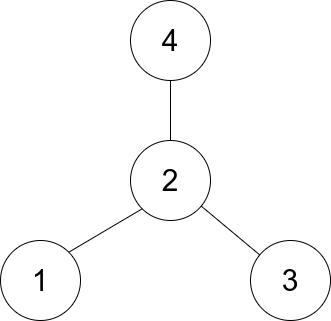1791. Find Center of Star Graph

There is an undirected star graph consisting of `n` nodes labeled from `1` to `n`. A star graph is a graph where there is one center node and exactly `n - 1` edges that connect the center node with every other node.

You are given a 2D integer array `edges` where each `edges[i] = [ui, vi]` indicates that there is an edge between the nodes `ui` and `vi`. Return the center of the given star graph.

Example 1:```Input: edges = [[1,2],[2,3],[4,2]]
Output: 2
Explanation: As shown in the figure above, node 2 is connected to every other node, so 2 is the center.
```

Example 2:

```Input: edges = [[1,2],[5,1],[1,3],[1,4]]
Output: 1
```

Constraints:

• `3 <= n <= 105`
• `edges.length == n - 1`
• `edges[i].length == 2`
• `1 <= ui, vi <= n`
• `ui != vi`
• The given `edges` represent a valid star graph.

1791. Find Center of Star Graph
``````struct Solution;

use std::collections::HashSet;

impl Solution {
fn find_center(edges: Vec<Vec<i32>>) -> i32 {
let mut hs: HashSet<i32> = HashSet::new();
for edge in edges {
let u = edge;
let v = edge;
if !hs.insert(u) {
return u;
}
if !hs.insert(v) {
return v;
}
}
0
}
}

#[test]
fn test() {
let edges = vec_vec_i32![[1, 2], [2, 3], [4, 2]];
let res = 2;
assert_eq!(Solution::find_center(edges), res);
let edges = vec_vec_i32![[1, 2], [5, 1], [1, 3], [1, 4]];
let res = 1;
assert_eq!(Solution::find_center(edges), res);
}
``````